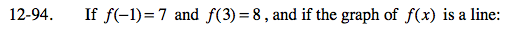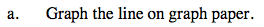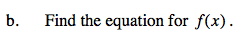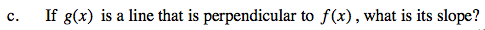### Home > CAAC > Chapter 12 > Lesson 12.4.3 > Problem12-94

12-94.f(-1) = 7 represents the point (-1, 7).
Graph this point and the point corresponding to f(3) = 8 in order to sketch the line.Use the y = mx + b format where m = slope (growth) and b = y-intercept (starting point).

Find the growth and substitute one of the coordinates of a point in the problem and solve for b.A line g(x) that is perpendicular to f(x) has a slope that is the negative reciprocal of the slope of the line f(x).